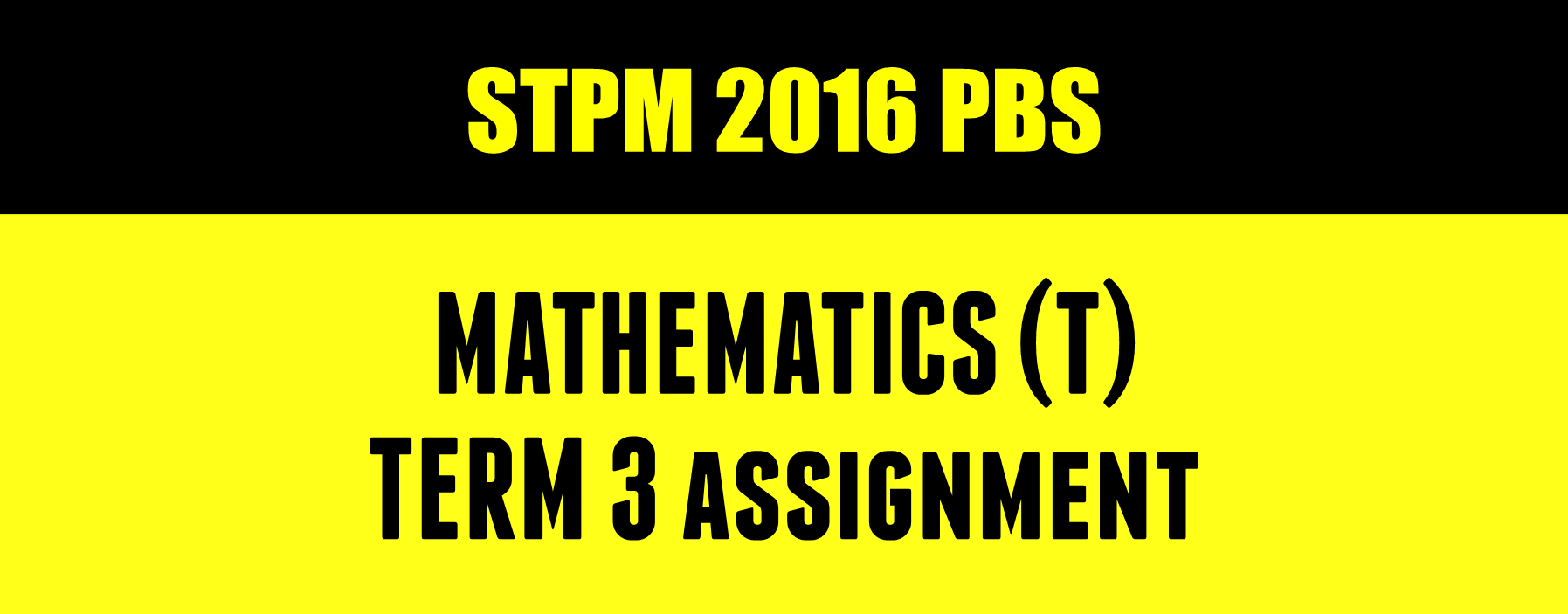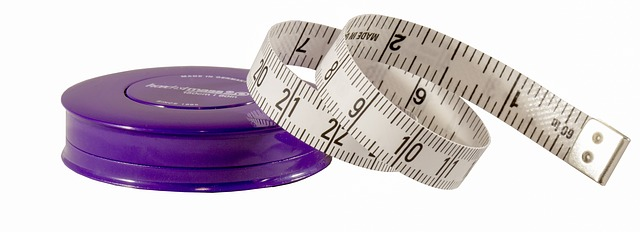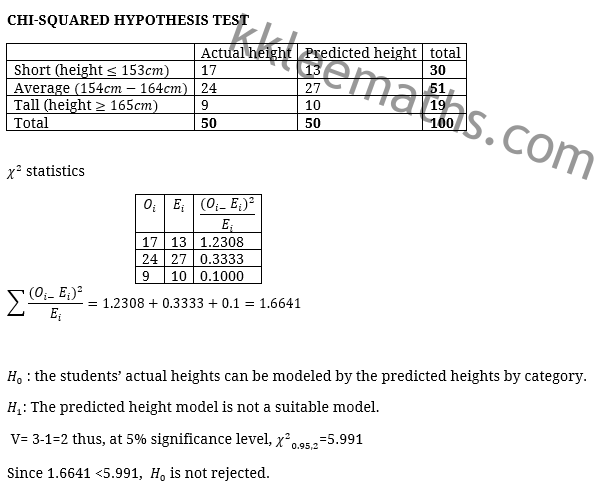#### kklee@kkleemaths.com#### Tuition Timetable

Check the latest timetable of my tuition classes.

#### Resources

Complete Resources about past year questions are here.

#### Ai Tuition

Visit Ai Tuition Website for more subjects tuition.

VIEW ALL SERVICES

Discussion –

20

Discussion –

20

# STPM Mathematics (T) Term 3 AssignmentSTPM 2016 Term 3 MT Coursework Explained
24
JUNE, 2016
Sampling
Confidence Interval
Hypothesis Testing
I will start posting Mathematics (T) coursework sample answer again this term. Only sample solution for mathematical part will be posted. Thanks
Parents often wonder how tall their kids would be. Would they be short, average, or tall? Is height inherited?

1 (a) Select a random sample of 50 upper secondary students of the same gender from your school. Describe how to ensure the randomness of your sample.
(b) Record the heights of the students and their parents in centimeters.

2 Determine suitable confidence intervals for the mean height of the students using a normal distribution. Interpret your results.Photograph by Johanna84 via Pixabay
The height, in cm, of a person can be predicted from one’s parents’ heights using the following formulae:
For girl: 0.5(father’s height + mother’s height) – 6.35
For boy: 0.5(father’s height + mother’s height) + 6.35
3 (a) Calculate the predicted heights of the students based on of the formulae given above.
(b) Classify the predicted heights into three categories (short, average and tall).

4 (a) Carry out a suitable hypothesis test to determine whether the students’ actual heights can be modeled by the predicted heights by category.
(b) What can you say about the formula given?
(c) Comment on whether the height of a person is inherited from one’s parents.Knowledge.
Photograph by jarmoluk via Pixabay
Sample solution will be posted once i discussed the solution in class. If you are my students and you need the solution asap, Whatsapp me! So that i can plan about the date to discuss the questions.

Here are our most recent updates posts

- Feel free to check it out -#### KK LEE

KK LEE has been a STPM Mathematics tuition teacher since June 2006, but his love of Maths dates back to at least 1999 when he was Form 4. KK LEE started teaching in 2006 at Pusat Tuisyen Kasturi. He was known as "LK" when he was teaching in PTK. After teaching STPM Mathematics for 8 years in PTK, he joined Ai Tuition Centre in 2014. Over the years he has taught Mathematics (T), Mathematics (S), Mathematics (M), Additional Mathematics.

1.Teacher for the intro,
What should we have in intro?

2.Teacher can i have the solution ASAP because i need to hand in this week.
Tq..

•ok. Posting

3.Sir, can I know how to make sentence for the question 4 (b)?

4.•You can check the first pic

5.how to know that the confidence interval is 95 percent?

•95% is just a sample. You can take any confidence level.

6.Teacher,can you give me the sample answer for this assignment?I neeeded to hand in this week,hope you can email to me.Thank you.

•Hi. The sample solution is given above. You can refer the two pictures attached.

7.Sir, is it possible to reject H0 ? I calculated my X2 cal is 11.922, is it too big?

•Yes

8.May I know the test statistics is mean-mean(predicted height of short group)？or mean-mean(short group)

•Which test statistic do you mean

9.sir, may i ask how to find the critical value at 8% of significance level?

•Which test are you referring?

10.Sir, may I know how to ensure the randomness of the sample. (The Question in 1.a)

•Prepare a box with balls with numbers

Pick a ball

11.Sir, can I know how to find the predicted height?

•